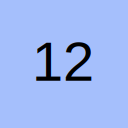# If then else if VBA

• I am trying to get the below statement work in VBA, but I always get the following error message: "Else without if"
[vba]
If Trim(.getstring(8, 49, 4)) = "" Then RSR_OBJECT!ACTDEPTTIME = Null
Else If .getstring(8, 49, 4) = 2400 Then RSR_OBJECT!ACTDEPTTIME = 23 & ":" & 59
Else if .getstring(8, 49, 4) <> 2400 Then
RSR_OBJECT!ACTDEPTTIME = .getstring(8, 49, 2) & ":" & .getstring(8, 51, 2)
End If
[/vba]
Thanks for any help
Johan

• Re: If then else if VBA

Try these see if they help -

jiuk
[vba]Option Explicit

Sub Version1()
If Trim(.getstring(8, 49, 4)) = "" Then
RSR_OBJECT!ACTDEPTTIME = Null
Else
If .getstring(8, 49, 4) = 2400 Then
RSR_OBJECT!ACTDEPTTIME = 23 & ":" & 59
Else
If .getstring(8, 49, 4) <> 2400 Then
RSR_OBJECT!ACTDEPTTIME = .getstring(8, 49, 2) & ":" & .getstring(8, 51, 2)
End If
End Sub
[/vba]
[vba]
Sub Version2()
If Trim(.getstring(8, 49, 4)) = "" Then
RSR_OBJECT!ACTDEPTTIME = Null
ElseIf .getstring(8, 49, 4) = 2400 Then
RSR_OBJECT!ACTDEPTTIME = 23 & ":" & 59
ElseIf .getstring(8, 49, 4) <> 2400 Then
RSR_OBJECT!ACTDEPTTIME = .getstring(8, 49, 2) & ":" & .getstring(8, 51, 2)
End Sub
[/vba]

• Re: If then else if VBA

If you're curious as to why you receive the "Else without If" error, it lies in VBA's limited support for the shorthand If. Specifically, a shorthand If statement (one where the body of the If is contained on the same line as the If itself) cannot contain any ElseIf or Else sections:

The first line of the second to code groups acts as a shorthand If, then VBA doesn't know what to do with the subsequent Else lines.

• Re: If then else in VBA

Appologies for the late reply but I have been struggling with this issue for the whole weekednd and beyond.
Root-cause turned out to be an End If that was positioned at the wrong place.
I also found out that that negation "ElseIf .getstring(8, 49, 4) <> 2400 Then ..." is not required afterall.
Bottomline is that my program does what it is supposed to do now.

Many thanks for all your input.
Johan